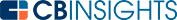# How to Calculate Nominal GDPWhen we refer to the term gross domestic product (GDP), we’re generally referring to nominal GDP. It’s also known as the current dollar GDP because it’s calculated using the current prices. Let’s take a closer look at what nominal GDP is and how to calculate it.

## What is nominal GDP?

GDP is an instrumental metric that governments and policymakers look at to gauge a country’s economic health. GDP can be nominal or real.

Nominal GDP is a measure of a country’s economic output calculated using current prices and without adjusting for inflation. Therefore, the increase and decrease in prices resulting from inflation or deflation are factored into the nominal GDP.

When comparing GDPs, ensure that you compare either two nominal values or two real values. Comparing a country’s nominal GDP with another country’s real GDP can give you an inaccurate calculation of the difference in growth between the countries.

## Nominal GDP vs. real GDP

Real GDP is a measure of a country’s economic output after adjusting the current prices for inflation. It offers better insight into a country’s long-term economic performance. This is because nominal GDP doesn’t tell us if the GDP increased as a result of higher production or inflation.

Economists prefer real GDP over nominal GDP because it offers a clearer picture of an economy’s growth. Real GDP eliminates the effect of inflation or deflation. This allows economists and investors to get a real sense of economic growth.

## Measuring nominal GDP

There are multiple methods for measuring nominal GDP. The most commonly used are the expenditure approach, the income approach, and the GDP deflator approach. Let’s take a look at how to use each method to calculate nominal GDP.

## Expenditure approach

The expenditure approach is the most widely used method for measuring nominal GDP. It’s measured as:

Y = C  + I  +  G  + (X – M)

Where:

• C = consumer spending – the total consumption of the households in an economy
• I = investment – investment by businesses on capital goods and inventory
• G = government spending – government spending on goods and services, excluding transfer payments
• X = exports – the total amount of goods and services produced in the country that were purchased in another country
• M = imports – the total amount of goods and services produced in another country that were purchased in the country for which we want to measure the GDP

## Income approach

Nominal GDP can also be measured using the earnings from resources used for production. It’s measured as:

Y = w  +  i  +  r  + p

Where:

• w = wages – the total wages earned from labor
• i = interest – the interest generated from capital
• r = rent – the rent earned on land
• p = profit – the profit earned by entrepreneurs

## GDP deflator approach

A GDP deflator tracks the changes in the price of a country’s output over a certain time. It can be used to convert real GDP to nominal GDP and vice versa.

To compute the GDP deflator, you need to assume a base year where nominal and real GDP are equal. Assign a value of 100 to this year and prepare a schedule that enlists nominal and real GDPs up to the current year.

For instance, let’s take a simplified example where a country produces a single product.You can use the following formula for calculating nominal GDP when you have the real GDP and GDP deflator:

Nominal GDP = real GDP x GDP deflator

Note that to calculate nominal GDP using the GDP deflator approach, you will need data on real GDP and the price change.

Nominal GDP, though less preferred by economists and investors, does have its uses. For instance, a country’s debt-to-GDP ratio will always use nominal GDP. This is because a country’s debt is always expressed in nominal terms. Using real GDP here would be technically incorrect.

Of the methods discussed above, the expenditure method is the most widely used. Note that only the final output of goods and services is measured while measuring the GDP. Intermediate goods are not added to avoid double-counting.

Several economists have criticized GDP’s use as a proxy for economic growth. GDP measurement doesn’t include the unorganized sector’s contributions to the economy and ignores business-to-business activities. However, GDP continues to be widely used across the globe as a measure of a country’s economic growth.

You may also like# www.cbinsights.com